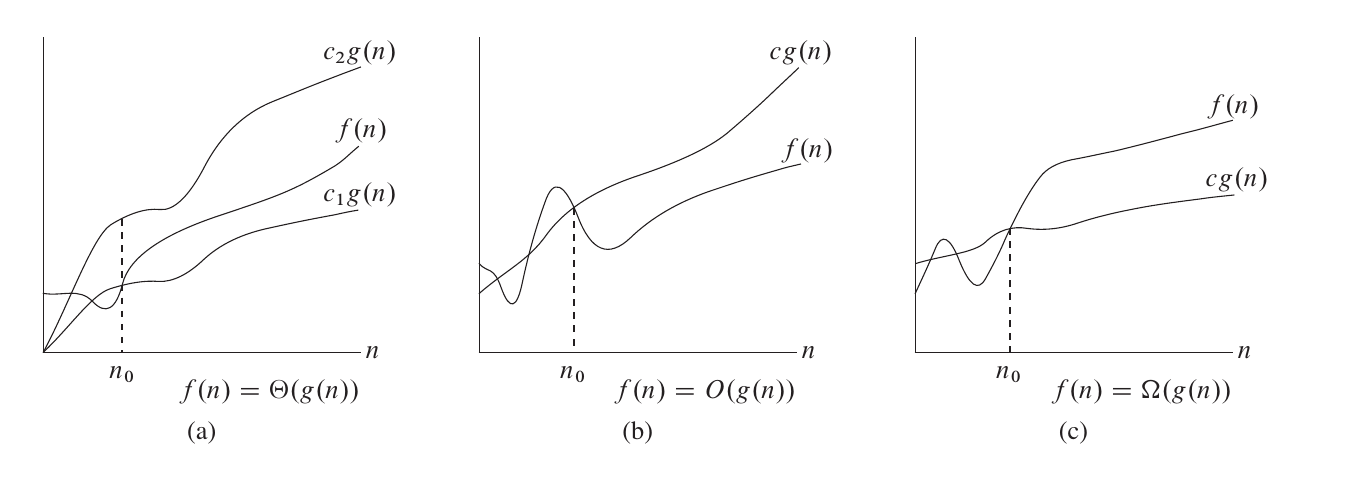02.算法复杂度

# 算法复杂度 #

## 1. 算法复杂度简介 #

「算法分析」的目的在于改进算法。

• 事后统计：将两个算法各编写一个可执行程序，交给计算机执行，记录下各自的运行时间和占用存储空间的实际大小，从中挑选出最好的算法。
• 预先估算：在算法设计出来之后，根据算法中包含的步骤，估算出算法的运行时间和占用空间。比较两个算法的估算值，从中挑选出最好的算法。

• 排序算法中：n 表示需要排序的元素数量。
• 查找算法中：n 表示查找范围内的元素总数：比如数组大小、二维矩阵大小、字符串长度、二叉树节点数、图的节点数、图的边界点等。
• 二进制计算相关算法中：n 表示二进制的展开宽度。

## 2. 时间复杂度 #

### 2.1 时间复杂度简介 #

• 基本操作 ：算法执行中的每一条语句。每一次基本操作都可在常数时间内完成。

 1 2 3 4 5  def algorithm(n): fact = 1 for i in range(1, n + 1): fact *= i return fact 

### 2.2 渐进符号 #

「渐进符号」 实际上是专门用来刻画函数的增长速度的。简单来说，渐进符号只保留了 最高阶幂，忽略了一个函数中增长较慢的部分，比如 低阶幂系数常量。因为当问题规模变的很大时，这几部分并不能左右增长趋势，所以可以忽略掉。

#### 2.2.1 $\Theta$ 渐进紧确界符号 #

$\Theta$ 渐进紧确界符号： 对于函数 $f(n)$ 和 $g(n)$，$f(n) = \Theta(g(n))$。存在正常量 $c_1$、$c_2$ 和 $n_0$，使得对于所有 $n \ge n_0$ 时，有 $0 \le c_1 \cdot g(n) \le f(n) \le c_2 \cdot g(n)$。

#### 2.2.2 $O$ 渐进上界符号 #

$O$ 渐进上界符号： 对于函数 $f(n)$ 和 $g(n)$，$f(n) = O(g(n))$。存在常量 $c$，$n_0$，使得当 $n > n_0$ 时，有 $0 \le f(n) \le c \cdot g(n)$。

$\Theta$ 符号渐进地给出了一个函数的上界和下界，如果我们只知道一个函数的上界，可以使用 $O$ 符号。

#### 2.2.3 $\Omega$ 渐进下界符号 #

$\Omega$ 渐进下界符号： 对于函数 $f(n)$ 和 $g(n)$，$f(n) = \Omega(g(n))$。存在常量 $c$，$n_0$，使得当 $n > n_0$ 时，有 $0 \le c \cdot g(n) \le f(n)$。### 2.3 时间复杂度计算 #

• 找出算法中的基本操作（基本语句）：算法中执行次数最多的语句就是基本语句，通常是最内层循环的循环体部分。
• 计算基本语句执行次数的数量级：只需要计算基本语句执行次数的数量级，即保证函数中的最高次幂正确即可。像最高次幂的系数和低次幂可以忽略。
• 用大 O 表示法表示时间复杂度：将上一步中计算的数量级放入 O 渐进上界符号中。

• 加法原则：总的时间复杂度等于量级最大的基本语句的时间复杂度。

• 乘法原则：循环嵌套代码的复杂度等于嵌套内外基本语句的时间复杂度乘积。

#### 2.3.1 常数 $O(1)$ #

$O(1)$ 只是常数阶时间复杂度的一种表示方式，并不是指只执行了一行代码。只要代码的执行时间不随着问题规模 n 的增大而增长，这样的算法时间复杂度都记为 $O(1)$。

 1 2 3 4 5  def algorithm(n): a = 1 b = 2 res = a * b + n return res 

#### 2.3.2 线性 $O(n)$ #

 1 2 3 4 5  def algorithm(n): sum = 0 for i in range(n): sum += 1 return sum 

#### 2.3.3 平方 $O(n^2)$ #

 1 2 3 4 5 6  def algorithm(n): res = 0 for i in range(n): for j in range(n): res += 1 return res 

#### 2.3.4 阶乘 $O(n!)$ #

 1 2 3 4 5 6 7 8 9  def permutations(arr, start, end): if start == end: print(arr) return for i in range(start, end): arr[i], arr[start] = arr[start], arr[i] permutations(arr, start + 1, end) arr[i], arr[start] = arr[start], arr[i] 

#### 2.3.5 对数 $O(log_2n)$ #

 1 2 3 4 5  def algorithm(n): cnt = 1 while cnt < n: cnt *= 2 return cnt 

#### 2.3.6 线性对数 $O(n log_2 n)$ #

 1 2 3 4 5 6 7 8  def algorithm(n): cnt = 1 res = 0 while cnt < n: cnt *= 2 for i in range(n): res += 1 return res 

### 2.4 最佳、最坏、平均时间复杂度 #

• 最佳时间复杂度：每个输入规模下用时最短的输入所对应的时间复杂度。
• 最坏时间复杂度：每个输入规模下用时最长的输入所对应的时间复杂度。
• 平均时间复杂度：每个输入规模下所有可能的输入所对应的平均用时复杂度（随机输入下期望用时的复杂度）。

 1 2 3 4 5 6 7  def find(nums, val): pos = -1 for i in range(n): if nums[i] == val: pos = i break return pos 

## 3. 空间复杂度 #

### 3.1 空间复杂度计算 #

#### 3.1.1 常数 O(1) #

 1 2 3 4 5  def algorithm(n): a = 1 b = 2 res = a * b + n return res 

#### 3.1.2 线性 O(n) #

 1 2 3 4  def algorithm(n): if n <= 0: return 1 return n * algorithm(n - 1) 

## 算法复杂度总结 #

「算法复杂度」 包括 「时间复杂度」「空间复杂度」，用来分析算法执行效率与输入问题规模 n 的增长关系。通常采用 「渐进符号」 的形式来表示「算法复杂度」。